﻿

### Maximal sector of analyticity for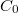$C_{0}$-semigroups generated by elliptic operators with separation property in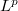$L^{p}$

#### Abstract

Analytic continuation of the$C_{0}$-semigroup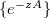$\{e^{-zA}\}$ on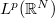$L^{p}(\mathbb{R}^{N})$ generated by the second order elliptic operator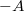$- A$ is investigated, where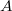$A$ is formally defined by the differential expression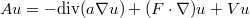$Au = -{\rm div}(a{\nabla}u) + (F\cdot{\nabla})u + Vu$ and the lower order coefficients have singularities at infinity or at the origin. \end

DOI Code: 10.1285/i15900932v33n2p65

Keywords: Second order linear elliptic operators in $L^{p}$; analytic $C_{0}$-semigroups; maximal sectors of analyticity

Full Text: PDF